[ IIMS 94 contents ]

## An introduction to vector quantisation

Y.-Kheong Chee, Harry Edgar, Doug Myers and Tom Docherty
Curtin University of Technology, Western Australia
Most multimedia applications make extensive use of video, audio, and images. As the storage space needed by such multimedia material increases, compression techniques are becoming an indispensable tool for these applications. This paper explains the basic principles of an image compression technique known as vector quantisation (VQ). The reasons why image compression is quickly becoming an indispensable tool in applications such as image databases and other multimedia packages are examined. The differences between scalar and vector quantisation are also explained. The basic operations of a vector quantiser are described and its advantages and disadvantages discussed. A brief update on current VQ research is given, showing how some of the VQ disadvantages can be minimised, and a comparison is made between a number of advanced image compression techniques, showing how VQ can be effectively integrated with other methods.

### Introduction

Image compression, which is the reduction of the quantity of data used to represent an image, is an important tool in multimedia applications. It is particularly indispensable in applications such as image databases and image transmission systems because of the significant reduction in data storage requirements it can provide. Image compression also paves the way for the new wave of so called photo-realistic multimedia applications. These applications try to meet the demand of users for more visually oriented software. It is no surprise then that image compression is a very active area of research. At the centre of all this activity is one of the most powerful and promising techniques: vector quantisation (VQ).

Vector quantisation can be considered to be a pattern matching process in which image blocks to be coded are matched with predefined blocks. The compression arises from the need to use less information to specify the predefined blocks. This paper is a tutorial presentation on vector quantisation. After introducing VQ, this paper will then delve into some more advanced VQ techniques. The following topics will be explored:

• The need for image compression;
• Differences between vector and scalar quantisation;
• Understanding of basic VQ and exposure to advanced VQ;
• Advantages and disadvantages: Why VQ is so effective and how even more potential can be tapped;
• Comparison between different compression techniques: compression ratio and quality.

### Why is image compression needed?

The fundamental goal of most image compression methods is reduced data transfer rate on a communication channel with minimum visual distortion. With the rapid advancement of communication technology in recent years, it has been argued that communication bandwidth is relatively inexpensive. However, with the increase in the amount of visually oriented applications, the amount of data to be handled and archived is also increasing substantially. As an example, as part of the Hubble Space Telescope project (White, 1992), around 600 GBytes of wide field photographic plates of the sky were digitised. Such large archives can only be distributed feasibly with an efficient image compression scheme. Other examples of applications that require intensive image processing and storage are scientific and engineering visualisation applications, which have become prevalent in recent years. Some of the primary uses of image compression are as follows (Gray et al., 1992):
1. Multimedia applications make extensive use of images and videos. With the advent of cheaper video technology, 24 bit colour video cards that display photo-realistic images are becoming popular in multimedia systems. The drawback is that 24 bit images are very large. For example, a 512 x 512 pixel 24 bit image occupies 768 kBytes. With 24 bit colour video, where 25 such image frames per second are required, a 60 minute video will need 69 Gbytes of storage. Such multimedia applications will only be feasible with compression methods.

2. The storage space saved from compression allows a greater number of images to be stored. In picture archival systems, for example, this is invaluable due to the enormous number of images to be stored. In applications such as medical imaging, this also means that more images can be stored on a convenient online storage before they must be archived to a large but remote offline storage (Gray et al., 1992).

3. Compression reduces the data rate, which means a reduction in transfer time when compressed images are transmitted across networks.

4. Some compression techniques can be extended to provide progressive image transmission. Here, an initial approximation of an image is sent first. The quality of this approximation is progressively improved while waiting for the user to decide whether to retain or reject the image.

5. Compression algorithms can be coupled with image processing codes so that images that are stored in a special structure can be more easily manipulated for tasks such as image enhancement and classification.

### What is scalar and what is vector quantisation?

Basically, the function of a quantiser is to map many input values or even a continuous function into a smaller, finite number of output levels. A scalar quantiser functions by mapping only one value at a time. On the other hand, a vector quantiser operates by mapping a block, or a vector, of values at a time. VQ is a much more complicated and lengthy process compared to scalar quantisation. Why is then VQ in such widespread usage? The answer lies in Shannon's rate distortion theory, which has shown that it is always possible to obtain better performance by coding vectors instead of scalars. Remarkably, this is even true if the data have been decorrelated or are independent.

The figure below shows an 8 x 8 grey scale image on the left and its equivalent digital representation on this right. Each number represents the intensity value of the corresponding pixel. The image representation is to be compressed by quantising its pixel values. Note that in reality, scalar quantisation is rarely done on an unprocessed image. In a compression method known as the differential pulse code modulation (DPCM) scheme (Gersho & Gray, 1992) for example, the pixel difference is coded. Here, however, the objective is to explain scalar quantisation.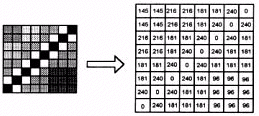Figure 1: A sample block of image pixels with its equivalent
numerical representation on the right

We assume that the image is to be compressed so that each pixel can be represented by just 2 bits instead of 8 bits. A quantiser can be described by its decision and reconstruction levels. For a variable X to be quantised, the quantisation rule operates as follows: if X falls in the decision region defined by xi and xi + 1 then it is mapped to or quantised to the corresponding reconstruction level yi. The layout of such a quantiser is shown below: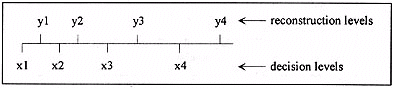Figure 2: A scalar quantiser

There are basically two types of scalar quantisers: uniform and non-uniform (Netravali & Haskell, 1989). The non-uniform quantiser can be optimised by using the Lloyd-Max quantiser algorithm (Netravali & Haskell, 1989). This quantiser has decision and reconstruction levels that yield the minimum distortion level for a given bit rate.

 i Decision levelxi Reconstruction levelyi Reconstruction levelindex 0 0 0 00 1 55 111 01 2 146 181 10 3 206 231 11 4 255 - -

Table 1: Decision and reconstruction levels of a scalar quantiser

With the example of coding the 8 x 8 image, a sub-optimal scalar quantiser described by the table below is used. The reconstruction levels are also assigned binary codes so that the effective amount of bits transmitted by the encoder is 2 bits per pixel (bpp).

For example, coding the first row of the image will yield the following output values: 111, 111, 231, 231, 181, 181, 231, and 0. These correspond to the following bit values: 01, 01, 11, 11, 10, 10, 11, and 00. A graph showing the decision and reconstruction levels along with a scatter plot of the grey value probabilities is displayed below.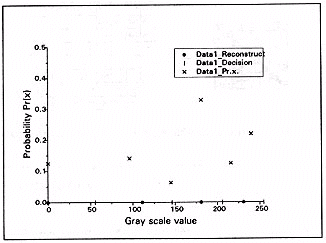Figure 3: Graph of a scalar quantiser's important data

The fidelity of the quantiser can be gauged by finding the mean square error (MSE) of the reconstruction. MSE is defined as: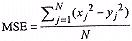A smaller MSE value indicates a higher reconstruction fidelity. In this example, the total number of pixels N is equal to 64. The MSE can be calculated to be 9583. A more popular measure of reconstruction fidelity is the power signal to noise ratio (PSNR). For a 256 level grey scale image, PSNR is defined as: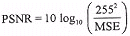A larger PSNR value indicates a higher reconstruction fidelity. For our example, PSNR is equal to 26.4 dB.

In vector quantisation, instead of coding individual values, a block of values, or a vector, is considered. For our example, we will take a block size of 2 x 2 pixels. The first step in VQ is to construct a codebook. This codebook consists of a set of different 2 x 2 pixel blocks or codewords, Xi', i = 1,..., Nc, where Nc is the number of codewords. The bit rate of the coder depends on the number of codewords in the codebook.

For example, if there are 8 codewords in the codebook, the bit rate is:

 log2 (number of codewords)block size = log2 8 bits4 pixels = 0.75 bit per pixel
After the codebook has been created, the encoding of the image begins. The operation is shown on the figure below. First, the image is divided into blocks of 2 x 2 pixels. Next, each image block X is compared to each of the entries Xi' in the codebook. The codeword that results in the least distortion is chosen and its index k is transmitted to the channel.

At the receiver, for each index k that is received, a table look up operation is performed. The codeword corresponding to k is retrieved from the codebook and is transmitted to be reconstructed. The reconstructed image is fully formed when all the indices have been received.

As before the fidelity of this codec (coder/decoder) can be evaluated by finding the PSNR. For illustrative purposes, an optimal codebook specific to the image to be coded will be used here. In practice it is virtually impossible to get such a codebook. The reason will become clear when codebook construction is explained later. The codebook is shown on the figure below. In examining the original 8 x 8 image, one can see that it has 9 different types of 2 x 2 blocks. Since our codebook will have only 8 codewords, codeword 111 is assigned to the combination between the last two 2 x 2 blocks shown on the figure below. Thus, we can expect fairly high reconstruction quality since only 2 blocks out of 64 are different from their original. The PSNR can be calculated to be 27.6 dB.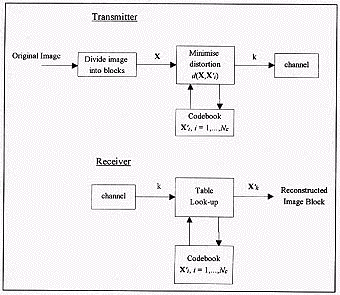Figure 4: Block diagram of a vector quantiser

Using scalar quantisation, 2 bpp are needed to yield a PSNR of 26.4 dB. VQ is more efficient, yielding 27.6 dB with only 0.75 bpp. It should be noted that this simple example only serves to illustrate that it is more efficient, in the sense of reducing data rate, to code vectors instead of scalars. The actual use of VQ is much more complicated and not as efficient as shown here.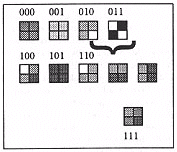Figure 5: Codebook

### Steps in VQ

#### Overview of steps involved

The following are the steps involved in basic VQ (refer to Figure 4 for a block diagram of the encoding and decoding operations):
1. Codebook generation
1. Construct an initial codebook
2. Improve the codebook by using an iterative algorithm

2. Encoding operations
1. Divide the image to be coded into vectors or blocks
2. Vector quantise each block by searching the codebook and finding the codeword that matches the image block with the least distortion
3. Transmit the index of the selected codeword

3. Decoding operations
1. At the decoder, retrieve the codeword that corresponds to the received index
2. The image is reconstructed when all the codewords have been retrieved.

#### Codebook generation

1. Constructing an initial codebook
As mentioned earlier, VQ is basically a pattern matching process. Each vector, for example an image block consisting of 2 x 2 pixels, is compared to a codebook that consists of a number of 2 x 2 codewords. The total number of codewords is Nc. The codebook can then be referred to as Xi', i = 1,2,..., Nc. Another important parameter to be defined is the distortion measure where d(X, X') denotes the distortion value between the original vector X and the codeword X'. m is the variable assigned to the length of the vector dimension. For codewords of size 2 x 2 pixels, m = 4. The most common distortion measure used is the mean square error; ie.,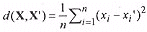The key to high image reconstruction quality is to have a codebook that matches the average image to be coded well. For this reason, codebook construction is a very important component of VQ.

Codebook design commonly requires a set of training images since accurate image statistics are usually unobtainable. Factors to be considered are the number of training images, the number of codewords in the codebook, and the dimension of the vector. Each of these represent a trade off between a pair of these parameters: image quality, bit rate, compression time, and memory requirements.

The construction of an initial codebook is an important procedure because a good initial codebook will help ensure that an efficient resulting codebook will be produced after the generalised Lloyd algorithm (Gersho & Gray, 1992) of the next step. There are a number of ways to construct an initial codebook (Netravali, 1989) (Gray et al., 1992) (Gersho & Gray, 1992):

1. A simple technique is to choose a subset of the training vectors. The selection criteria should filter out similar vectors or abnormal ones.

2. Another straightforward technique is to use a coarse scalar quantiser on the individual vector components. The resolution of the quantiser will be dependent on the number of resulting codewords. Such a codebook is also known as product codebook.

3. The splitting algorithm operates by adding codewords to a codebook until a sufficiently low distortion value is obtained. It starts by calculating the centroid of the entire training set. This codeword is then split to form a pair of codewords. The generalised Lloyd (GL) algorithm is then executed to optimise these two codewords. This algorithm will be described in the next section. This process continues in a similar manner, which is: given K codewords, generate 2K codewords by perturbing each codeword and then execute the GL algorithm to optimise the new codewords. Note that this algorithm produces an initial codebook and also improves it, while the previous two methods can only create an initial codebook.
A valid question is how many training images should be used in codebook creation. This problem has its roots in information theory (Gray, 1984): for the training images to be representative of the source, the source will have to be stationary (the statistics of the source are the same in all parts of the source) and ergodic (a typical sample function can statistically represent the source). Neither of these assumptions are true when image sources are considered; however, these assumptions can provide a quick approximation.

The practical solution is as follows: a reasonable amount of training images is first used to create the codebook. The robustness of the codebook is tested by using it to code a number of images that were not in the training set. If the results produced are satisfactory (the distortions produced are close to the average distortion value that was used for creating the codebook), it suggests that the number of training images used is sufficient.

2. Codebook improvement: the generalised Lloyd algorithm
Once an initial codebook has been constructed, the next step is to run an iterative codebook improvement algorithm to optimise it. A vector quantiser can be considered optimal if it minimises the average distortion for the given parameters of dimension, codebook size, and number of training images. The most common codebook improvement algorithm is the generalised Lloyd (GL) algorithm, which is commonly but less appropriately referred to as the LBG (Linde Buzo Gray) algorithm. With a given initial codebook Xi', i = 1,2,..., Nc, a distortion measure d(X, X'), and average distortion D, the algorithm proceeds as follows:

1. Using the distortion measure, map each vector of the training images into its closest code word. This mapping is known by a number of names: the minimum distortion, Voronoi, or nearest neighbour mapping. This mapping will create a decision region for each codeword. The decision region of a codeword encloses the image vectors that have been mapped to it. When an iteration of the entire training set has been processed, the average distortion is calculated. If this is less than or equal to the given threshold D, convergence has been achieved and the algorithm stops. Otherwise, it proceeds to the next step.

2. For each decision region, its corresponding codeword is replaced with a new vector that minimises the distortion for that region. If the MSE distortion measure is used, the new codeword can be calculated by finding the centroid of the training vectors contained in the decision region. The centroid is the mean of the vector components. Consider, for example, a decision region in a codebook using 2 dimensional vectors. The existing codeword is [2 1]. The training vectors that have been mapped to this region are [2 3] and [1 1]. The centroid of these two vectors is (m, the dimension of the codeword, is 2):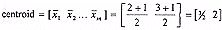As can be seen above, each component of the centroid vector is found by calculating the mean of the corresponding components in the training vectors. The algorithm is then repeated by returning to step 1.

An important point to note is that the GL algorithm can only be optimum for the initial codebook the algorithm started with. In other words, only a local optimum can be obtained. Other codebook improvement techniques aim to provide a more global optimum by introducing randomness in the codebook shaping process so that there is less dependency on the initial codebook. A family of such algorithms in based on a method called stochastic relaxation (Gersho & Gray, 1992).

#### Encoding operations

After a locally optimal codebook has been generated, the next step is to divide the image to be coded into the correct block or vector dimension. Refer to Figure 4. This block size is dependent on the block size of the codewords. Each image vector is then vector quantised by searching the codebook for its nearest neighbour. The nearest neighbour is the codeword that matches image vector with the least distortion. The index of the nearest neighbour codeword is then retrieved and transmitted to the receiver channel.

The encoding operations can be very lengthy, depending on the vector dimension and the size of the codebook. The search technique is known as exhaustive searching since every codeword is compared to the image vector. For the MSE distortion criterion, each comparison can be mathematically described as: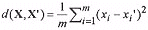where d(X, X') is the distortion measure and m is the vector dimension. For example, if the image vector to be coded, xi, is [3 1] and the codeword being compared to is [1.5 2],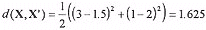The nearest neighbour codeword for an image vector is found when its d(X, X') value is lower than any other codeword in the codebook.

#### Decoding operations

The decoding operations are fairly simple in VQ. The decoder also has a copy of the codebook used by the encoder. For each index received from the transmitter, the decoder retrieves its corresponding codeword. This is a simple table look up operation. The retrieved image block is then used a part of the reconstructed image. These simple operations permit VQ to operate at very high decompression rates.

The advantages of VQ are as follows:
1. Very high compression ratio and quality can be achieved: theoretically the Shannon's rate distortion bound can be achieved with a large vector dimension. This rate distortion bound provides an unsurpassable bit rate for any given average distortion. The bit rate of a vector quantiser is given by

2.  R = log2 Ncn bits / pixel

3. where Nc is the number of codewords in the codebook and n is the dimension of the codeword. Theoretically, the performance can be close to the rate distortion bound as n tends to infinity. In practice, of course, the codebook storage and search complexity would make a design with very large n unfeasible. On the other hand, fairly good performance can be achieved with modest vector dimensions.

4. Image decompression is very quick since it consists mainly of a table look up operation. In a typical type of image database application, an image is compressed once when it is first archived into the system. It is subsequently decompressed many times each time the user retrieves the image. For such applications, the benefits of a fast decompression time far outweighs inconvenience of slow compression.

5. Since VQ is a technique that has only relatively recently been popularised, there is still much room for improvement. This is manifested by the high concentration of research in this area. Another reason for its popularity is that it can be relatively easily combined with other techniques. A later section will expand on some powerful VQ enhancements.

6. Because vectors, and not scalars, are being coded, it is possible to have fractional bit allocation. For example, scalar PCM is restricted to having a bit rate of at least 1 bit per sample while a k dimensional VQ can have a bit rate that is a multiple of 1/k bits per sample (Gray, 1984).

7. We can incorporate image processing techniques such as enhancement and classification into the encoder so that these tasks can be achieved together with image compression. For some very good examples, refer to Gray et al's (1992) excellent article.

While it enjoys certain advantages, VQ has its heavy share of disadvantages too. Most of these stem from the need to use a codebook. The disadvantages become more and more imposing as the vector dimension increases or the size of the codebook increases.
1. The encoding process can be very computationally intensive and slow. This complexity increases exponentially with the vector dimension. On the other hand, a performance drop will occur with small vector dimensions since the non-stationarity nature of the image cannot be taken into account and the bit rate will be high.

2. Memory storage requirement for the codebook also increases rapidly with increasing vector dimension and codebook size. For real time VLSI implementation, this is also limited by the cost and size of ROM (Read Only Memory) modules.

3. Codebook generation is a very lengthy process, especially with large vector dimensions and codebooks. However, since codebook generation is done offline, this disadvantage is somewhat allayed.

4. Images that are different from the training images cannot be coded with high fidelity. For example, if the training set consists mainly of head and shoulder type images, it will not be effective for coding an image of a building. To ensure that the training set is a good representation, a sufficiently large number of appropriate training images should be used.

5. As with many other compression schemes that divide the image into sub-blocks, the blocking effect is apparent in low bit rate VQ. This is a disturbing artefact that can be seen as visible discontinuities across block boundaries.

The codec for basic full search VQ previously described is actually not used much in advanced image coding applications. Its quality is poor for coding at low bit rates because complexity and memory usage increase exponentially with vector dimension. On the other hand, at high bit rates, complexity and memory increase tremendously with bit rate. This section outlines a number of improvements that have been added to the basic VQ. Further details can be obtained from (Gersho & Gray, 1992).

1. More effective codebook generation
The generalised Lloyd algorithm is improved with methods such as stochastic relaxation (codebook design is offline and an improvement of 0.5 dB in SNR can be effected) and fuzzy clustering. The codebook produced by such techniques are less dependent on the initial codebook when compared to the conventional method.

2. Faster encoding algorithm
This is accomplished in several ways. In tree structured VQ and lattice VQ, a structural constraint is imposed on the codebook. Such methods yield below optimal results but the reductions in complexity and encoding time can be enormous. Research has also been done to apply different search strategies. For example, the partial distance encoder progressively calculates the distortion value so that it can eliminate a large proportion of the codewords prematurely.

3. Generating more efficient codebooks
As mentioned earlier, an efficient codebook reflects accurately the properties of the images to be coded. One of the grave shortcomings with basic VQ is that the lack of constraints makes it very difficult to represent an "average* image successfully. Enhanced VQ try to more accurately pinpoint commonly used codewords by imposing constraints on the image structure. When the vector blocks to be coded have a smaller variance, it is also possible to reduce the size of the codebook.

One example is classified VQ, whereby a simple scheme is used to classify image vectors into edges and uniform blocks. A codebook is used for the edge codewords and a different one for the uniform block codewords.

Another example is the hybrid method that performs a discrete cosine transform (DCT) on the image block before vector quantising it. The DCT decorrelates the image block and helps to build a more focussed VQ codebook.

4. Higher compression rates
This objective is also related to higher efficiency codebook construction. Some methods used in current research include variable block sizes, larger vector dimensions, and smaller codebooks. Variable block sizes permit variable bit rates. A side benefit is that it enables the perceived quality to be approximately constant across the entire image. Also, this is beneficial with the advent of packet switching networks (eg. Broadband ISDN), whereby the data rate is not constant because data are only sent when necessary. Another efficient technique is entropy constrained VQ, in which the entropy cost of the codeword is included into the cost function, instead of just the MSE.

A VQ system is adaptive if its codebook or encoding rule changes to match observed local statistics of the input sequence. Examples are mean adaptive VQ, switched codebook adaptation, variable bit rate VQ, and adaptive codebook VQ.

6. Reduction of the blocking artefact
This can be accomplished in a number of ways. One of the most effective is to use side match classified VQ (Kim, 1992). The encoder selects a codebook, from a number of different ones, according to the nearest row and column of the neighbouring blocks.

7. Progressive image transmission
Progressive image transform (PIT), also mentioned in the section "Why is image compression needed?", is a very powerful bandwidth reduction technique. Reduction is accomplished in two ways: compression of data and premature termination of transmission. The two dominant techniques in VQ are multistage VQ, where the residual error is coded each stage, and pyramidal VQ, where the image is coded hierarchically.

### Comparisons

This section briefly compares the performance of various VQ schemes, as well as the JPEG compression standard. The difficulty with any such comparisons has to do with the choice of comparison criteria. There is no ideal compression scheme. The best one can hope for is an ideal for a very specific application. This is because each application has its own requirements. It may be that it has to adopt a widely used compression technique, in which case JPEG may be the best solution. In yet another application, the user may desire moderate image quality but very low bit rate. In such a case, T. Senoo and B. Girod's (1992) technique, lattice VQ for entropy coding of image sub-bands, might be the best solution.

For the comparison here, the target images to be encoded are natural images, as opposed to computer produced graphics. The criteria chosen here are the very general ones: objective image quality and bit rate. The compression is made on how well a method can compress the Lena image. Lena is a 512 x 512 pixel 256 grey scale image that has become a very popular choice for simulations. The original image is shown below.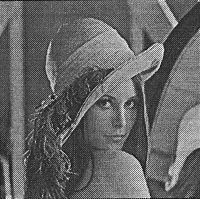Figure 6: The Lena image used for comparison

The issue of subjective image quality is a very difficult one to solve in image processing. The most common measures of fidelity so far have been based on error norms such as the mean squared error (MSE). However, there is a human in the image compression loop, and the human observer is the final judge of the reconstructed image's fidelity. The problem with using criteria such as MSE is that such measures do not accurately reflect the perceptual notions of visual fidelity. An example of this observation can be seen by adding noise to an image.

If the noise is added randomly, the degradation will not be as noticeable compared to the degradation when the same amount noise is concentrated on a mall area of the image. Some suggested ways to overcome this problem is to introduce a weighting system into the distortion measure and to consider the modulation transfer function (MTF) of the human visual system. Two weaknesses with such solutions are that there is no commonly agreed method and there is a high computational cost. In addition, subjective quality often varies depending on the image compression's application. The current favoured solution is to publish a compressed image along with an original one. This is not done here due to the lack of space. However, they can be seen by looking up the appropriate journals referenced in this paper.

Most of compression methods analysed here involve some form of VQ but the fractal, wavelet, and JPEG compression methods do not. They are included on the basis that they are very popular in image compression research or yield very high compression ratios. The techniques listed here can be considered to be representative of state of the art methods in image compression. A more rigorous representation is very difficult to obtain since researchers do not publish their PSNR results and some do not simulate on the Lena image.

 Method Bit rate(bpp) PSNR(dB) Darragh 0.26 31.27 Jacquin 0.68 27.7 Riskin 1 0.32 29.29 Riskin 2 0.32 30.92 Antonini 1 0.37 30.85 Antonini 2 0.21 29.11 Huang 0.20.250.30.40.50.75 29.70330.92531.8433.04733.98535.369 Fisher 0.2175 30.71 Kim 0.6390.4090.310.481 37.2335.2834.0435.98 Senoo 0.136 30.91 Andrew 0.80.40.2 363330 Gharavi 0.55 33.95 Ngan 0.45 34.25 JPEG 0.250.5 30.8134.69

Table 2: Comparison between various compression techniques
1. Darragh and Baker (1988): fixed distortion, variable rate sub-band coding.
2. Jacquin (1990): fractal block coding
3. Riskin (quoted from Antonini, 1992): a) full search VQ and b) pruned tree structured VQ
4. Antonini et al. (1992): wavelet transform with multi-resolution codebook VQ a) high rate version and b) low rate version
5. Huang et al. (1992): prioritised (partition priority coding) DCT using multiple distribution entropy coding (MDEC)
6. Fisher et al. (1992): fractal image coding
7. Kim and Modestino (1992): Adaptive entropy coded sub-band coding with VQ
8. Senoo and Girod (1992): Lattice VQ for entropy coding of image sub-bands (orthogonal lattice and optimum bit allocation rule)
9. Andrew et al. (1993): wavelet transform (12 tap minimum time width)
10. Gharavi and Tabatabai (1988): Sub-band coding
11. Ngan et al. (1991): Hybrid classified VQ and DCT with HVS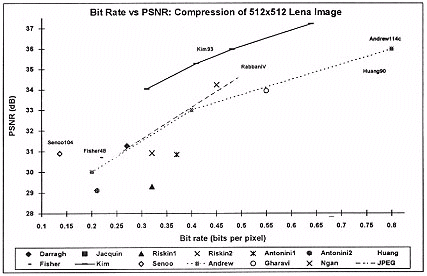Figure 7: Graph showing comparison between various compression techniques

From table 2 and the graph on figure 7, it can be seen that full search VQ performs poorly. This confirms the observation made earlier that basic VQ is not an efficient compression method. However, when VQ is combined with other compression methods, the performance increase is significant. Outstanding methods are Kim and Modestino's and Senoo and Girod's compression schemes. However, one drawback with these methods is that the complexities and encoding times are usually quite imposing.

It can also be observed that the JPEG compression method performs very favourably. Another strong motivation to use JPEG is that it is a popular image compression standard and can be implemented rather easily. There are already many companies producing hardware for performing JPEG compression. However, JPEG is fast reaching its limitations, whereas it is still possible to improve on VQ based techniques.

### Conclusion

Vector quantisation is a very promising image compression technique. Its strength is derived from basic information theory: rate distortion theory maintains that higher performance can be achieved by coding vectors instead of scalars. This attribute enables VQ based compression to produce very high image quality with low bit rates. Other VQ advantages include its fast decompression speed, fractional bit rate characteristic, and the ease in which it can be combined with other compression methods. It is also important to understand VQ's share of disadvantages. This knowledge along with an understanding of other compression techniques will allow one to combine VQ favourably into a hybrid compression scheme.

VQ is currently a highly active area of research, arguably the most busy for image compression. The reason behind this is that there is still much room for improvement and new VQ based techniques have been shown to be able to outstrip other compression techniques significantly.

### References

Andrew, J. P., Ogunbona, P. O. & Paoloni, F. J. (1993). Image coding using DCT and wavelet techniques. MCAT.

Antonini, M., Barlaud, M., Mathieu, P. & Daubechies, I. (1992). Image coding using wavelet transform. IEEE Trans. Image Proc., 1(2), 205-20.

Darragh, J. & Baker, R. (1988). Fixed distortion, variable rate sub-band coding of images. Proc. SPIE, 1001, 979- 990.

Fisher, Y., Jacobs, E. W. & Boss, R. D. (1992). In Image and Text Compression (ed J. A. Storer), Fractal image compression using iterated transforms. pp. 35-61.

Gersho, A. & Gray, R. M. (1992). Vector quantisation and signal compression, 1st edition. Kluwer Academic Publishers.

Gharavi, H. & Tabatabai, A. (1988). Sub-band coding of monochrome and color images. IEEE Trans. Circuits Syst., 35, 307-14.

Gray, R. M. (1984). Vector quantisation. IEEE ASSP Magazine, 1, 4-29.

Gray, R. M., Cosman, P. C. & Riskin, E. A. (1992). In Image and Text Compression (ed J. A. Storer), Image compression and tree-structured vector quantisation, pp. 3-34.

Huang, Y., Dreizen, H. M. & Galatsanos, N. P. (1992). Prioritised DCT for compression and progressive transmission of images. IEEE Trans. Image Proc., 1(4), 477-87.

Kim, T. (1992). Side match and overlap match vector quantizers for images. IEEE Trans. Image Proc., 1(2), 170-85.

Kim, Y. H. & Modestino, J. W. (1992). Adaptive entropy coded sub-band coding of images. IEEE Trans. Image Proc., 1(1), 31-48.

Netravali, X& Haskell, B. (1989). Digital pictures: Representation and compression, 1st edition. Plenum Press.

Ngan, K. N., Koh, H. C. & Wong, W. C. (1991). Hybrid image coding scheme incorporating human visual system characteristics. Optical Engineering, 30(7), 940-6.

Rabbani, M. & Jones, P. W. (1991). Digital image compression techniques, 1st edition, chap. 12. SPIE.

Senoo, T. & Girod, B. (1992). Vector quantisation for entropy coding of image sub-bands. IEEE Trans. Image Proc., 1(4), 526- 32.

Wallace, G. K. (1991). The JPEG still picture compression standard. Commun. ACM, 34(4), 3 144.

White, R. (1992). High performance compression of astronomical images. Internal report, University of Colorado.

 Authors: Mr. Y.-Kheong Chee, PhD Student, Curtin University of Technology, GPO Box U 1987, Perth WA 6001. Tel: (home) 313 1352 Email: scheeyk@cc.curtin.edu.au Dr. T. Harry Edgar, Senior Lecturer, School of Electrical and Computer Systems Engineering, Curtin University of Technology, GPO Box U 1987, Perth WA 6001. Tel: 470 2428 Email: tedgarth@cc.curtin.edu.au Dr. Doug G. Myers, Head of Department, Computer Systems Engineering, Curtin University of Technology, GPO Box U 1987, Perth, WA 6001. Tel: 470 2428 Email: tmyersdg@cc.curtin.edu.au Tom Docherty, Senior Lecturer, School of Electrical and Computer Systems Engineering, Curtin University of Technology, GPO Box U 1987, Perth WA 6001. Tel: 470 2428 Email: tdocherty@cc.curtin.edu.au Please cite as: Chee, Y.-K., Edgar, T. H., Myers, D. and Docherty, T. (1994). An introduction to vector quantisation. In C. McBeath and R. Atkinson (Eds), Proceedings of the Second International Interactive Multimedia Symposium, 88-98. Perth, Western Australia, 23-28 January. Promaco Conventions. http://www.aset.org.au/confs/iims/1994/bc/chee.html

[ IIMS 94 contents ] [ IIMS Main ] [ ASET home ]
This URL: http://www.aset.org.au/confs/iims/1994/bc/chee.html
© 1994 Promaco Conventions. Reproduced by permission. Last revision: 3 Feb 2004. Editor: Roger Atkinson
Previous URL 29 Apr 2000 to 30 Sep 2002: http://cleo.murdoch.edu.au/gen/aset/confs/iims/94/bc/chee.html